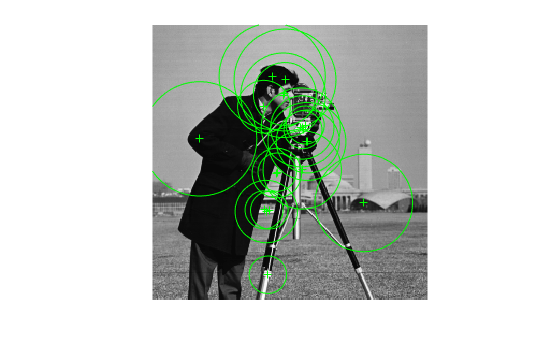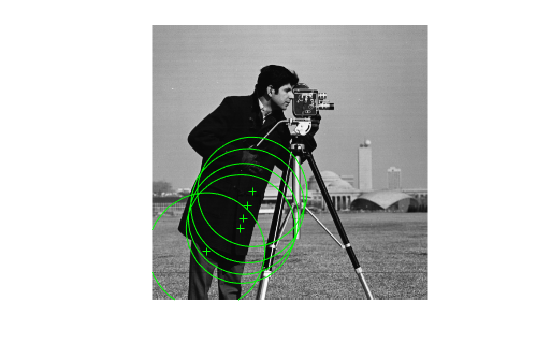# detectKAZEFeatures

Detect KAZE features

## Syntax

``points = detectKAZEFeatures(I)``
``points = detectKAZEFeatures(I,Name=Value)``

## Description

example

````points = detectKAZEFeatures(I)` returns a `KAZEPoints` object containing information about KAZE keypoints detected in a 2-D grayscale image. The function uses nonlinear diffusion to construct a scale space for the given image. It then detects multiscale corner features from the scale space.```
````points = detectKAZEFeatures(I,Name=Value)` specifies options using one or more name-value arguments in addition to the previous syntax. For example, `detectKAZEFeatures(I,Threshold=0.003)` sets the threshold to exclude less significant local extrema to `0.003`.```

## Examples

collapse all

`I = imread("cameraman.tif");`

Detect KAZE points in the image.

`points = detectKAZEFeatures(I);`

Plot the 20 strongest points.

```imshow(I) hold on plot(selectStrongest(points,20)) hold off```Read an image into the workspace.

`I = imread("cameraman.tif");`

Detect KAZE features in the image.

`points = detectKAZEFeatures(I);`

Select and display the last 5 points detected.

```imshow(I); hold on; plot(points(end-4:end)); hold off;```## Input Arguments

collapse all

Input image, specified as a 2-D grayscale image.

Data Types: `single` | `double` | `int16` | `uint8` | `uint16` | `logical`

### Name-Value Arguments

Specify optional pairs of arguments as `Name1=Value1,...,NameN=ValueN`, where `Name` is the argument name and `Value` is the corresponding value. Name-value arguments must appear after other arguments, but the order of the pairs does not matter.

Example: `detectKAZEFeatures(I,Threshold=0.003)` sets the threshold to exclude less significant local extrema to `0.003`.

Method to compute conductivity, specified as `"region"`, `"sharpedge"`, or `"edge"`. The computation is based on first-order derivatives of a layer in scale space.

MethodSelected Features
`"region"`Large regions. Uses the Perona and Malik conductivity coefficient, ```1/(1 + dL^2/k^2)```.
`"sharpedge"`High-contrast edges. Uses the Perona and Malik conductivity coefficient, `exp(-|dL|^2/k^2)`.
`"edge"`Smoothing on both sides of an edge rather than across it. Uses the Weickert conductivity coefficient.

Local extrema, specified as a scalar greater than or equal to `0`. Increase this value to exclude less significant local extrema.

Multiscale detection factor, specified as a positive integer. Increase this value to detect larger features. To disable multiscale detection, set `NumOctaves` to `1`. When you set the value to `1`, the function performs the detection at the scale of the input image. Recommended values are between 1 and 4.

Scale levels, specified as an integer in the range [3,10]. Increase this value to achieve smoother scale changes. Increasing this value also provides additional intermediate scales between octaves.

Rectangular region size for corner detection, specified as a 4-element vector in the format [y x width height]. The [y x ] values are measured from the upper left corner of the rectangle.

## Output Arguments

collapse all

KAZE points, returned as a `KAZEPoints` object. The object contains information about the feature points detected in the 2-D grayscale input image.

 Alcantarilla, P.F., A. Bartoli, and A.J. Davison. "KAZE Features." ECCV 2012, Part VI, LNCS 7577. 2012, p. 214